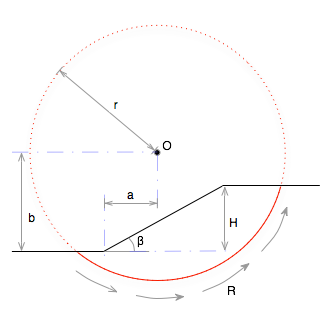•http://facebook.com/
•https://www.google.com/accounts/o8/id
•https://me.yahoo.com
COST (GBP)8.000.000

# slope safety factor

The stability (safety factor) of a undrained cut or built slope.
Controller: CodeCogsC++

## Slope Safety Factor

 doubleslope_safety_factor( double radius double a double b double H double slope double soil_density double soil_c )
Computes the safety factor of a relatively short cut or built slope, where undrained conditions exist along a potential circular failure plane. The mode of failure is shown in the figure below, and is described by the selection of a,b and the radius r that intersect the slope and describe the slip plane.The factor of safety is calculated by taking moments about the center of the slip circle (O): where the resistance moment is caused by the shear resitance of the soil along the length of the potential failure plane and the activation moment is caused by the weight of the soil above the presumed slip plane.

Private Section

## Example

#include <stdio.h>
#include <codecogs/engineering/geotechnics/slope_safety_factor.h>
int main()
{
double soil_c=45;
double soil_density=1900;
double H=9;  // height of bank
double slope=90;
double a=6;    // horizontal distance to toe of slope

// Find the vertical position of the circle that gives the lowest factor of safety
for (double b=10; b < 40; b += 1)
{
double R=sqrt(b*b+a*a); // assume minimum radius to toe of slope
double F=Engineering::Soil::slope_safety_factor(R, a, b, H, slope, soil_density, soil_c);

}

return 0;
}
Output:
TODO: Need to compute

### Parameters

 radius The radius r of the slip circle. Note the radius must be sufficient to reach below the toe of the slope and beyond the top of the slope. [m] a The horizontal offset from the toe of the slope to the center of the slip cicle. [m] b The vertical offset from the toe of the slope to the cetner of the slip circle. [m] H The height of the slope. [m] slope The angle of the slope. [degrees] soil_density The average density of the soil within the slope. [kg/m3] soil_c The natural cohesion (base shear strength) of the soil, . [N/m^2]
##### Source Code

Source code is available when you buy a Commercial licence.

Not a member, then Register with CodeCogs. Already a Member, then Login.